In this section and the next, we present the standard cash flow and pricing calculations for a mortgage-backed security consisting of a single tranche. In the final section, we show how this single-tranche approach is easily generalized for a multi-tranche security.

MONTHLY MBS COUPON AND WAC

The typical Canadian balloon mortgage has fixed-level payments made monthly and a mortgage rate compounded semi-annually. In order to calculate MBS cash flows, we convert the annual MBS coupon and the annual weighted average mortgage rate to monthly rates: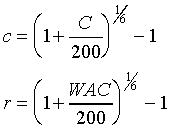MONTHLY PAYMENT

In the calculations that follow, we wish to price the mortgage-backed security at some time k=0. This can be at issue, or at some later date in the security's life. The first step is to calculate the scheduled monthly payment at the pricing date k=0. All calculations are per dollar of remaining principal balance at the time of pricing, Bo.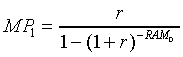where

RAM0=remaining average amortization at time of price r=weighted average mortgage coupon, expressed on a monthly basis.

When calculating MP1, it is assumed that all mortgages in the single-tranche pool have the same mortgage rate and amortization schedule.

When a mortgage in the pool liquidates, there is one less mortgage contributing to the monthly payment on the mortgage-backed security. Thus, the monthly payment must be reduced to account for liquidations. If q denotes the constant fraction of mortgages that liquidate each month, then 1-q denotes the fraction remaining. Thus, the monthly payment after k months is: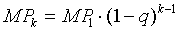This could also be calculated recursively as: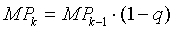As long as there are a reasonable number of mortgages remaining in the pool, liquidations do not substantially affect the term or amortization of the security. This is because the liquidation of one mortgage has no affect on the cash flows of the mortgages that remain in the pool. The situation is reversed in the case of partial prepayments, which leave the monthly payment unchanged, but reduce the term and amortization of the mortgage.

SCHEDULED REMAINING PRINCIPAL BALANCE

The scheduled remaining principal, denoted SRPk, is the previous month's balance less scheduled principal payments. In the standard formulas, it is calculated as follows. Last month's balance is grossed-up by r, the monthly weighted average mortgage rate. The monthly payment of scheduled interest and principal, MPk, is then subtracted from this grossed-up figure to obtain SRPk: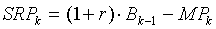where

MPk=MPk-1·(1-q)
r=monthly weighted average mortgage rate
B0=1
BM=0

REMAINING PRINCIPAL BALANCE

The remaining principal balance for month k can be calculated by applying the prepayment rates, q and p, to the scheduled remaining principal, SRPk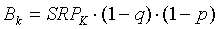MONTHLY CASH FLOW

The monthly cash flow received by the MBS holder, CFk, is calculated as follows: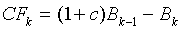The valuation standards do not consider penalty interest payments. To incorporate penalties into MBS pricing, a term denoting the monthly penalty interest payment, PIPkwould be added to the right hand side of the above equation. This calculation, included in the PCBOND Manager Software, is shown in Appendix 4

Date Published: March 31, 2018